# Taylor Series for ln(1+x): How-to & Steps

Lesson Transcript
Instructor: Gerald Lemay

Gerald has taught engineering, math and science and has a doctorate in electrical engineering.

In this lesson, we show how to find the Taylor series for ln(1+x). This is a series evaluated about a specific point so we also look at the interval of convergence about this point.

## The Taylor Series Expression

The Taylor series represents a function of x where the function is evaluated about a value, a. As an infinite sum, we have this: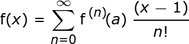The Σ symbol means ''summation.'' We evaluate the expression to the right of Σ for n = 0, and then this result is added to the expression evaluated for n = 1, n = 2, and so on all the way to n = ∞. Let's expand this sum into the first 5 terms:An error occurred trying to load this video.

Try refreshing the page, or contact customer support.

Coming up next: Representing the ln(1-x) Power Series: How-to & Steps

### You're on a roll. Keep up the good work!

Replay
Your next lesson will play in 10 seconds
• 0:02 The Taylor Series Expression
• 0:36 Taylor Series Steps
• 5:31 Solution
• 5:57 Interval of Convergence
Save Save

Want to watch this again later?

Log in or sign up to add this lesson to a Custom Course.

Timeline
Autoplay
Autoplay
Speed Speed

## Taylor Series Steps

Here are the steps for finding the Taylor series of ln(1 + x).

#### Step 1: Calculate the first few derivatives of f(x).

We see in the formula, f(a). This is f(x) evaluated at x = a. Then, we see f '(a). This is the first derivative of f(x) evaluated at x = a.

Thus, the first step is to calculate derivatives of the function, f(x) = ln(1 + x).

The derivative of ln(1 + x) = 1/(1 + x) times the derivative of 1 + x. The derivative of 1 + x = 0 + 1 = 1. So far we have this: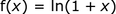And this: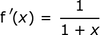To find the next derivative, note 1/(1 + x) is the same as (1 + x)-1. The derivative of (1 + x)-1 is (-1)(1 + x)-2, which equals -1/(1 + x)2. Thus: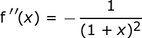Differentiating again, we get this: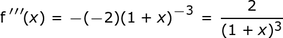Let's do one more.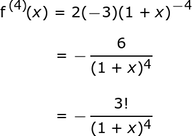We write 6 as 3!. The exclamation point is the factorial, meaning 3(2)(1). Note we could have written 2 as 2! because 2! = 2(1). By the way, 1! is 1 and 0! is also equal to 1.

#### Step 2: Evaluate the function and its derivatives at x = a.

Take each of the results from the previous step and substitute a for x. For f(x) = ln(1 + x) we get f(a) = ln(1 + a). For the first derivative: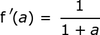Likewise, for each of the derivatives we evaluated earlier: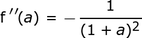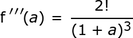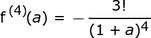#### Step 3: Fill in the right-hand side of the Taylor series expression.

We now build the Taylor series from this:This gives us this: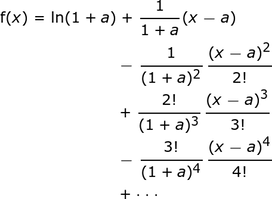In the third line, we see 2! over 3!. This can be simplified because 2!/3! = 2(1)/3(2)(1) = 1/3. Likewise, in the next line, 3!/4! = 1/4. Our expression becomes this: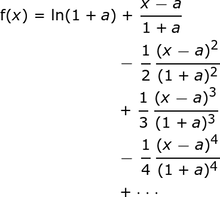#### Step 4: Write the result using a summation.

Having a summation of a general term will be useful when determining the interval of convergence, or the set of x-values where a series converges. After the ln(1 + a) term, we see the numerator with an (x - a) term raised to increasingly higher powers. Likewise, the denominator has a (1 + a) term that grows with increasing powers. There is also an integer in the denominator that increases linearly. Lastly, the sign of the terms alternates.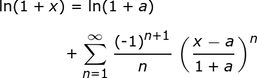You might try expanding this sum to convince yourself if it really does represent the terms of our expression.

## Solution

So the Taylor series of ln(1 + x) about the point a is this:## Interval of Convergence

Let's find the interval of convergence. The general term, An, is this: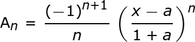Replacing n with n + 1 we get An+1: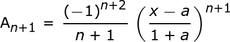The ratio test says a series will converge provided: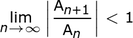To unlock this lesson you must be a Study.com Member.
Create your account

### Register to view this lesson

Are you a student or a teacher?

### Unlock Your Education

#### See for yourself why 30 million people use Study.com

##### Become a Study.com member and start learning now.
Back
What teachers are saying about Study.com
Create an account to start this course today
Used by over 30 million students worldwide